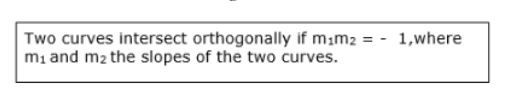# Show that the following curves intersect orthogonally at the indicated points :

Question:

Show that the following curves intersect orthogonally at the indicated points :

$x^{2}=y$ and $x^{3}+6 y=7$ at $(1,1)$

Solution:

Given:

Curves $x^{2}=y \ldots$ (1)

$\& x^{3}+6 y=7 \ldots(2)$

The point of intersection of two curves $(1,1)$

Solving (1) \& (2), we get,

First curve is $x^{2}=y$

Differentiating above w.r.t $x$,

$\Rightarrow 2 x=\frac{d y}{d x}$

$\Rightarrow \frac{d y}{d x}=2 x$    .....(3)

Second curve is $x^{3}+6 y=7$

Differentiating above w.r.t $x$,

$\Rightarrow 3 x^{2}+6 \cdot \frac{d y}{d x}=0$

$\Rightarrow \frac{d y}{d x}=\frac{-3 x^{2}}{6}$

$\Rightarrow \frac{d y}{d x}=\frac{-x^{2}}{2}$

$\Rightarrow m_{2}=\frac{-x^{2}}{2} \ldots(4)$

Substituting $(1,1)$ for $m_{1} \& m_{2}$, we get,

$m_{1}=2 x$

$\Rightarrow 2 \times 1$

$m_{1}=2 \ldots(5)$

$m_{2}=\frac{-x^{2}}{2}$

$\Rightarrow \frac{-1^{2}}{2}$

$m_{2}=-\frac{-1}{2} \ldots(6)$

when $m_{1}=2 \& m_{2}=-\frac{-1}{2}$$\Rightarrow 2 \times \frac{-1}{2}=-1$

$\therefore$ Two curves $x^{2}=y \& x^{3}+6 y=7$ intersect orthogonally.